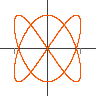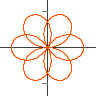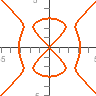Plots implicit and algebraic curves like `x^2-y^3`. Type the equation of the curve into box at the bottom or select one from the list on the right. You can click and drag the mouse to shift the screen, and use a mouse wheel to zoom, arrow keys also shift and +, -, PageUp, PageDown zoom. Right clicking brings up a menu with other display options.

The algorithm works by evaluating the equation at every point on the screen, if there is a sign change then a point is plotted. Speed is achieved by using the fasteval Jep extension, this is an evaluator optimized for evaluation over the reals, which is nearly ten times faster than the standard Jep evaluator. You can compare speeds by unticking the Fast method option in the right click menu.

## Jep plotting appsGraphs
`y=a x^2+b x+c`
JavaScript Version
Java VersionParametric
`x=cos(t), y=sin(t)`
JavaScript Version
Java VersionPolar
`r = cos(3 th/2)`
JavaScript Version
Java VersionComplex
`(z+a)/(z+b)`
JavaScript VersionImplicit
`x^2+y^2=1`
JavaScript Version
Java Version